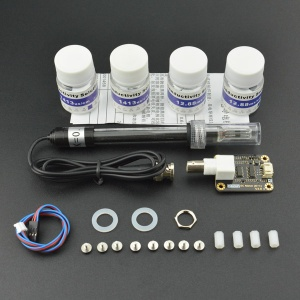## 概述

• 想DIY一个电导率计吗？还在为没有一款价格低廉、使用方便的电导率计而烦恼吗？对电导率计的来龙去脉感到陌生？不过在DFRobot，陌生感很快就会消失。我们最新推出了一款模拟电导率计，具有连线简单、方便实用等特点。按照示意图连线后，再通过程序控制，就可以非常方便的测量溶液的电导率了。
• 电导率是物质传送电流的能力，是电阻率的倒数。在液体中常以电阻的倒数——电导来衡量其导电能力的大小。水的电导是衡量水质的一个很重要的指标，它能反映出水中存在的电解质的浓度。根据水溶液中电解质的浓度不同，则溶液导电的程度也不同。在国际单位制中，电导率的单位称为西门子/米（S/m），其它单位有：S/m，mS/cm，μS/cm。换算关系为：1S/m=1000mS/m=1000000μS/m=10mS/cm=10000μS/cm。

## 技术指标

• 工作电压：+5.00V
• PCB尺寸：45mm×32mm
• 测量范围：1ms/cm--20ms/cm
• 适用温度：5-40℃
• 精度：<±10%F.S.(具体精度取决于你的校准溶液精度)
• PH2.0接口（3脚贴片）
• BNC接口型电导率电极（电导池常数为1）
• 电导电极线缆长度：大约60厘米
• 防水型DS18B20温度传感器
• 电源指示灯

## 电极尺寸图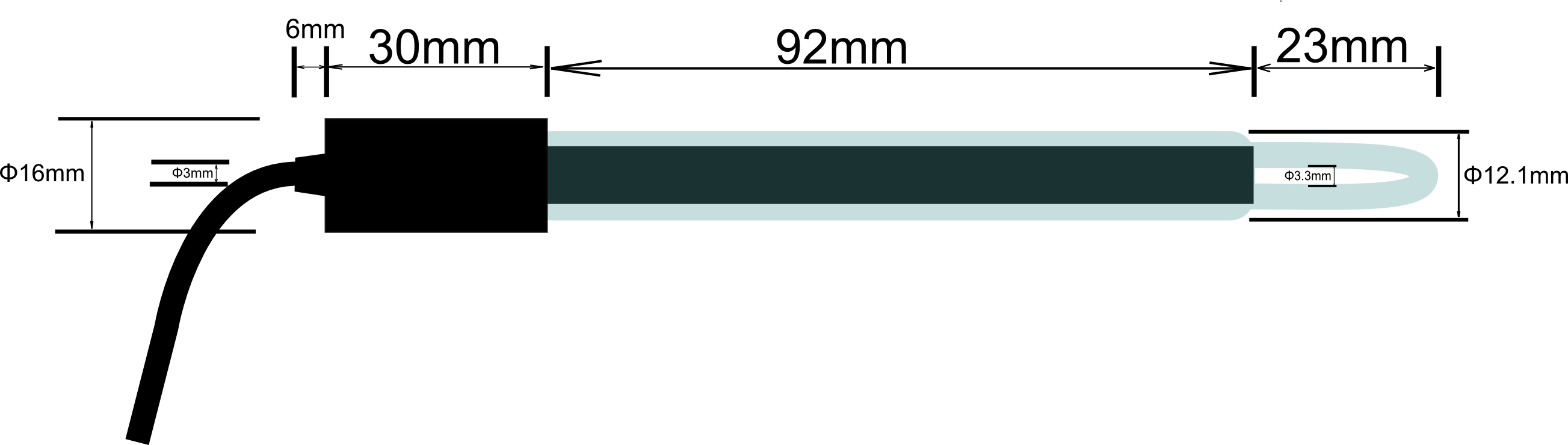## 电导率计的使用（初级）

### 连接图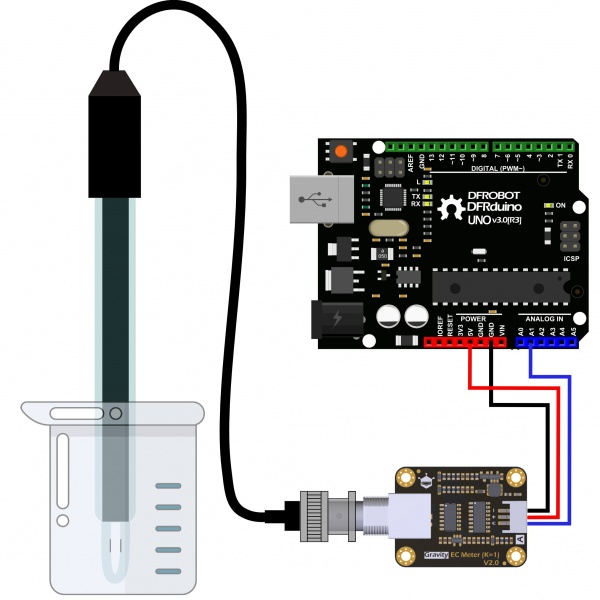### 使用步骤

• 请使用外接电源，使得EC Meter的供电电压接近+5.00V，电压越准，精度越高。

• 每测量不同的溶液前，需要使用清水清洗电导率电极和温度传感器，以防止示数不准和溶液污染。建议使用去离子水清洗。

• 测量溶液的电导率时，请务必确保温度电极插进被测溶液中，并且用电导电极搅拌溶液，使得电导电极的导电部分充分接触溶液。待温度值和电导率值稳定后，即可读取所需的值。受溶液极化影响，测量高电导率的溶液时，电导率示数会出现一定范围内的抖动。电导率越高，抖动越厉害。

(1)将各个设备按照图示方式连接，即：电导率电极连接到EC Meter电路板的BNC接口，然后用模拟连接线，将EC Meter电路板连接到Arduino主控器的模拟口1。然后把防水型DS18B20温度传感器连接到可插拔传感器转接器的接线端子，然后用数字连接线，连接到Arduino主控器的数字口2。对Arduino主控器供电后，可以看到EC Meter电路板的蓝色指示灯变亮。

(2)对Arduino主控器烧写样例代码。

(3)打开Arduino IDE的串口监视器，此时能输出一些参数，如电压值、温度值，并且提示没有溶液。(4)将电导率电极和温度传感器插入校准溶液中，可以测量该溶液的电导率值。搅拌溶液，待示数稳定。如果示数接近标准溶液瓶身所标的值，则可以投入使用。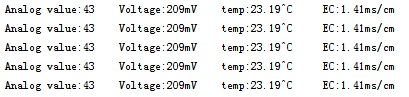## 样例代码

``````    #include <OneWire.h>

#define StartConvert 0

const byte numReadings = 20;     //the number of sample times
byte ECsensorPin = A1;  //EC Meter analog output,pin on analog 1
byte DS18B20_Pin = 2; //DS18B20 signal, pin on digital 2
unsigned int AnalogSampleInterval=25,printInterval=700,tempSampleInterval=850;  //analog sample interval;serial print interval;temperature sample interval
byte index = 0;                  // the index of the current reading
unsigned long AnalogValueTotal = 0;                  // the running total
unsigned int AnalogAverage = 0,averageVoltage=0;                // the average
unsigned long AnalogSampleTime,printTime,tempSampleTime;
float temperature,ECcurrent;

//Temperature chip i/o
OneWire ds(DS18B20_Pin);  // on digital pin 2

void setup() {
// initialize serial communication with computer:
Serial.begin(115200);
// initialize all the readings to 0:
TempProcess(StartConvert);   //let the DS18B20 start the convert
AnalogSampleTime=millis();
printTime=millis();
tempSampleTime=millis();
}

void loop() {
/*
Every once in a while,sample the analog value and calculate the average.
*/
if(millis()-AnalogSampleTime>=AnalogSampleInterval)
{
AnalogSampleTime=millis();
// advance to the next position in the array:
index = index + 1;
// if we're at the end of the array...
// ...wrap around to the beginning:
index = 0;
// calculate the average:
}
/*
Every once in a while,MCU read the temperature from the DS18B20 and then let the DS18B20 start the convert.
Attention:The interval between start the convert and read the temperature should be greater than 750 millisecond,or the temperature is not accurate!
*/
if(millis()-tempSampleTime>=tempSampleInterval)
{
tempSampleTime=millis();
}
/*
Every once in a while,print the information on the serial monitor.
*/
if(millis()-printTime>=printInterval)
{
printTime=millis();
averageVoltage=AnalogAverage*(float)5000/1024;
Serial.print("Analog value:");
Serial.print(AnalogAverage);   //analog average,from 0 to 1023
Serial.print("    Voltage:");
Serial.print(averageVoltage);  //millivolt average,from 0mv to 4995mV
Serial.print("mV    ");
Serial.print("temp:");
Serial.print(temperature);    //current temperature
Serial.print("^C     EC:");

float TempCoefficient=1.0+0.0185*(temperature-25.0);    //temperature compensation formula: fFinalResult(25^C) = fFinalResult(current)/(1.0+0.0185*(fTP-25.0));
float CoefficientVolatge=(float)averageVoltage/TempCoefficient;
if(CoefficientVolatge<150)Serial.println("No solution!");   //25^C 1413us/cm<-->about 216mv  if the voltage(compensate)<150,that is <1ms/cm,out of the range
else if(CoefficientVolatge>3300)Serial.println("Out of the range!");  //>20ms/cm,out of the range
else
{
if(CoefficientVolatge<=448)ECcurrent=6.84*CoefficientVolatge-64.32;   //1ms/cm<EC<=3ms/cm
else if(CoefficientVolatge<=1457)ECcurrent=6.98*CoefficientVolatge-127;  //3ms/cm<EC<=10ms/cm
else ECcurrent=5.3*CoefficientVolatge+2278;                           //10ms/cm<EC<20ms/cm
ECcurrent/=1000;    //convert us/cm to ms/cm
Serial.print(ECcurrent,2);  //two decimal
Serial.println("ms/cm");
}
}

}
/*
ch=0,let the DS18B20 start the convert;ch=1,MCU read the current temperature from the DS18B20.
*/
float TempProcess(bool ch)
{
//returns the temperature from one DS18B20 in DEG Celsius
static byte data;
static float TemperatureSum;
if(!ch){
Serial.println("no more sensors on chain, reset search!");
ds.reset_search();
return 0;
}
Serial.println("CRC is not valid!");
return 0;
}
Serial.print("Device is not recognized!");
return 0;
}
ds.reset();
ds.write(0x44,1); // start conversion, with parasite power on at the end
}
else{
byte present = ds.reset();
for (int i = 0; i < 9; i++) { // we need 9 bytes
}
ds.reset_search();
byte MSB = data;
byte LSB = data;
float tempRead = ((MSB << 8) | LSB); //using two's compliment
}
return TemperatureSum;
}
``````

## 电导率计的使用（高级）

### 测量原理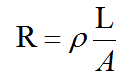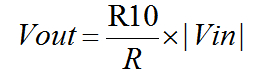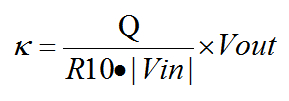## 注意事项

• 电导率电极有光亮和铂黑两种，镀铂黑的目的在于增加电极片的有效面积，减弱电极的极化，测量电导率较大的溶液，使用铂黑电极比较适宜。

• 铂黑电极的铂金片表面附着有疏松的铂黑层，应避免任何物体与其触碰，只能用去离子水进行冲洗，否则会损伤铂黑层，导致电极测量不准确。

• 如发觉铂黑电极使用性能下降，可依次使用无水乙醇和去离子水浸洗铂金片，特别是用户对测量精度要求较高时尤为重要。

• 铂黑电极的铂金片表面附着有疏松的铂黑层，在测量样品时有可能会吸附样品成分，在使用电极测量完毕后一定要及时冲洗电极。

• 电导率电极在放置一段时间或者使用一段时间后，其电极常数有可能发生变化。如用户对测量准确度要求较高，建议按照仪器说明书定期校正电极常数。